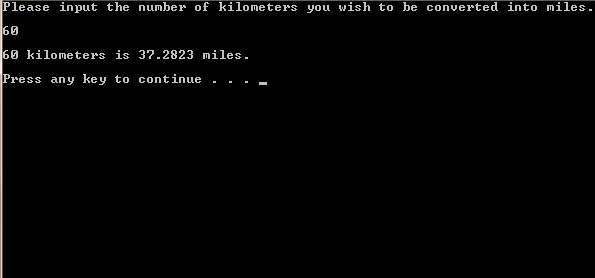# C / C++ Code / Program To Convert Kilometer Distance Into Miles

/* A program to convert (input) kilometers into miles. User is asked to enter the distance in kilometers & the program returns the corresponding miles value */

#include <iostream>
#include <cstdlib>
using namespace std;

int main()
{

// Declaring and initializing the variable
float k = 0;

// Prompting the user for input (in km).
cout << "Please input the number of kilometers you wish to be converted "
"into miles."
<< endl << endl;
cin >> k;

// Display number of kilometers in miles.
cout << endl
<< k << " kilometers is " << (k * 0.621371192) << " miles."
<< endl << endl;

system("PAUSE");
return EXIT_SUCCESS;
}

Screenshot:Screen Shot of the output of C / C++ Code / Program To Convert Kilometer Distance Into Miles